# I didn't expect that after reading this article, I immediately understood the principle of Vue data-driven view

2021-08-27 07:45:28

## Preface

​ Hello everyone , I am a `console No, log`, A sophomore college student , This article tells you to share vue The principle of data-driven view , Also mixed with the knowledge of template compilation . I will write this article very simply , believe me , You must understand .

​ In this article, I mainly focus on the needs 、 flow chart 、 The logic that explains the principle in the code , I don't have much to say. I'll start writing articles now .

## Go into the small knowledge points of the project

` Closure `

The scope is divided into ： Global scope and local scope

Variables in the local scope , After the execution of this scope method , Will be recycled by the garbage collection mechanism

``````function run() {
let a = 11
return a
}

let num = run() //  When the code is executed this time, it will be executed in run Method locally scoped variables a It will be disposed of by the garbage collection mechanism
let num2 = run() //  When executing this code , It will recreate a local scope , Generate a variable a, When the function is executed, it will be processed by the garbage collection mechanism again

Copy code ``````
``````function run () {
let a = 11
let b = 12

function asd() {
a = a+1
return a
}

return asd
}

let me = run() //  At this time, according to the original scheme ,run The variables in the local scope of will be in run After method execution , Will be recycled by the garbage collection mechanism , But found a The variable is used by another local scope , It will have an effect , Namely run Other variables under this local scope will be disposed of by the garbage collection mechanism ,a The variable will be saved in memory .
let num = me() //  The variables in memory will be read here a And add one to return , There will be a Destroy variables ？  The answer is not ,a Still in memory   here num The value of is  12
console.log(num);  // -- 12

let num2 = me() //  The print out here is 13, It's obvious , Called twice to return me Method uses the same memory address a Variable
console.log(num2); // -- 13

Copy code ``````

Here we mention that the parameters of a function also enjoy such rights , Functions declare variables , That is, declaring a variable in the local scope is just useless var let const These are just a few declaration marks , So let's look at the code

``````function run(a) {

function asd() {
a += 1
return a
}

return asd
}

let me = run(1)
let num = me()
console.log(num); // -- 2
let num2 = me()
console.log(num2); // -- 3

//  Let's not talk about the principle , It is also possible to express the parameters of the function .
Copy code ``````

`Object.defineProperty`

You can see this link directly Object.defineProperty() - JavaScript | MDN (mozilla.org), If you don't understand, let's see my explanation .

This method will directly define a new attribute on an object , Or modify the existing properties of an object , And return this object .

``````let obj = {}

Object.defineProperty(obj, 'a', {
value: '111',
})

console.log(obj); // -- { a : '111' }

Object.keys(obj).forEach(key => console.log(key)); // -- 'a'

//  This method will directly define a new attribute on an object , And you can set the value
Copy code ``````
``````let obj = {}

Object.defineProperty(obj, 'a', {
value: '111',
enumerable: false //  Here you can set whether this key can be enumerated , If it is false If we don't use for...in..., still Object.keys I won't read it out
})

console.log(obj); // -- { a : '111' }

Object.keys(obj).forEach(key => console.log(key)); // --  No printing

for (let key in obj) {
console.log(key); //  No printing
}

Copy code ``````
``````let obj = {}

Object.defineProperty(obj, 'a', {
value: '111',
writable: false //  Set whether this value can be modified , If it is false It cannot be modified
})

console.log(obj); // -- { a : '111' }

obj.a = '222'

console.log(obj) // -- { a : '111' }
Copy code ``````

What's written below get and set

get： Represents the function triggered when the attribute is used , The return value of the function is the value obtained when using

set： When setting a new value for this property , Trigger function , Function has an argument , Is the new value you set

``````//  The following picture is the print after the code block runs , It is found that the maximum stack memory is exceeded , Why is that
let obj = {
a: 11
}

Object.defineProperty(obj, 'a', {
get() {
console.log('obj.a It's used ');
return ' I am a a'
},
set(val) {
// console.log(val) // -- 12
obj.a = val; //  This is because , If you give it directly to obj.a  The assignment is equivalent to starting from one time set Method , Infinite trigger set It's going to burst
}
})

let a = obj.a // -- ‘ I am a a’

obj.a = 12
Copy code ``````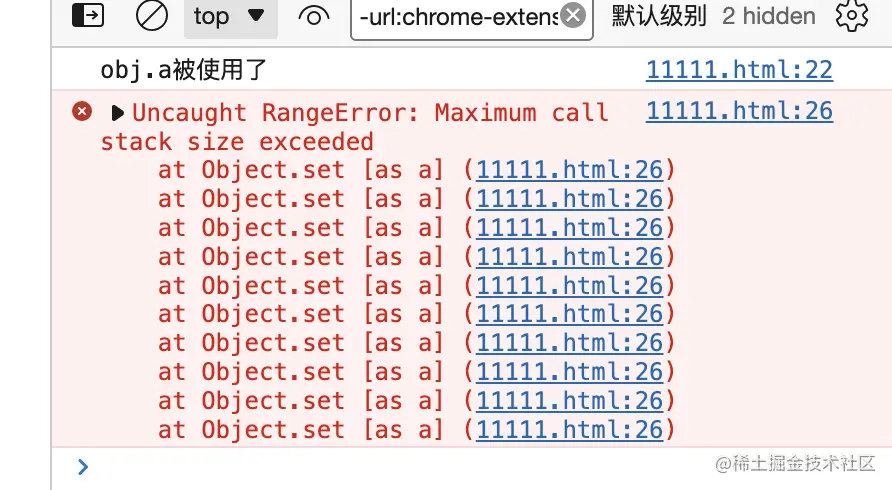How to solve this problem , Look at the following code

Solution 1

``````//  We can accept the new value we set through a variable ,get It also returns the variable that we set the new value

let obj = {
a: 11
}
let temp;

Object.defineProperty(obj, 'a', {
get() {
console.log('obj.a It's used ');
return temp
},
set(val) {
temp = val;
}
})

obj.a = 12

let as = obj.a
console.log('as The value of is ：', as);
//  Here are the results
Copy code ``````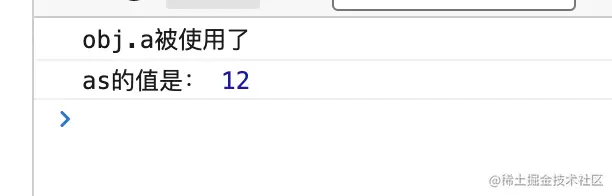Solution 2

Closure is used here , Remember we said that the parameter of the function actually declares a new variable , The parameter variable here is equivalent to the... In the code block above `temp` He acts as an intermediary

``````let obj = {
a: 11
}
function defineReactive(obj, key, value) {
Object.defineProperty(obj, key, {
get() {
return value;
},
set(newValue) {
value = newValue;
}
})
}

defineReactive(obj)
let as = obj.a
console.log(as);
obj.a = 22
console.log(obj);
Copy code ``````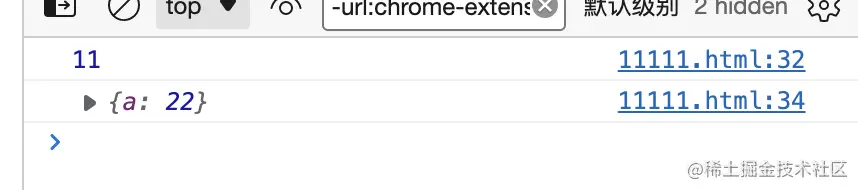` Design patterns -- Subscription publishing mode `

I think this article is well written , You can go and have a look Observer mode vs Publish subscribe mode - You know (zhihu.com) After reading the articles , Come back and see my explanation

The publish subscribe mode is actually one to many , Let's take a real-life example

「 There is a sales office , There is a boss in the sales office （dep） There are three salesmen in the sales office （watcher） Now Zhang San , Li Si , Wang Wu came to buy a house , They are respectively received by a sales counterpart , But now there is no house. Some sales asked her corresponding customers to go back and wait for notice ,` And then one day , The house has ,dep Inform each watcher Said there was a house ,watcher Inform all his corresponding customers that they have a house , When all customers hear the notice , Come and sell the house `, This example is the publish subscribe mode 」

## Build the base catalog

1. First we create a new folder `my-vue`（ my vue）
2. Let's execute `npm init -y` Initialize our project
3. Use `yarn add vite` install vite Environmental Science
4. Create a file at the root `index.js` and `index.html` And in html Write the words we want to see
5. Run on the command line `vite` So our project can run , Click the link to see that we are html The page written in

## Overall function

Because we just study data-driven views , So we only realize data Just the function of

## Theoretical knowledge

Vue use data Hijack combined publishers - Subscriber pattern To realize the data response , adopt Object.defineProperty ( Click me to view this attribute ) To hijack data setter,getter, Publish messages to subscribers when data changes , After receiving the message, the subscriber performs corresponding processing ..

## Theoretical realization

First, in the `observer` In the process of registration `get` Method , This method is used for 「 Rely on collection 」. There will be a... In its closure `Dep` object , This object is used to store Watcher Instance of object . Actually 「 Rely on collection 」 The process is to turn `Watcher` The instance is stored in the corresponding `Dep` Go to the object .`get` Methods can make the current `Watcher` object （Dep.target） Stored in its subs in （`addSub`） Method , When the data changes ,`set` Would call `Dep` Object's `notify` Method to notify it of all internal `Watcher` Object for view update .

In my own words, it is , I passed ` Object.defineProperty` This api to `data` All the attributes in , add get（ Use it ）,set（ Change it ） How to trigger .`Compiler` In this class , What we use on the page data We have bound a variable inside `watcher` Listening in , And this monitor , We `Dep` In this general manager , When the page data changes , Trigger set Method , Notify our corresponding Manager , Let him tell everyone `watcher` monitor , Trigger their own page update method .

## Data driven prologue

1. stay `index.js` write in vue Class

• Save the to mount dom Elements , By default, we bind id by app Of dome Elements
• Execute the incoming data function , Get the data object he returns
``````class Vue {
constructor(option) {
this.\$el = option.el ?
document.querySelector(option.el)
:
document.querySelector('#app')

this.\$data = option.data()

}
}

Copy code ``````
2. We want to directly use this visit data Data in

• stay Vue Create... In this class `_proxyData` Method , Receive a parameter （data）
• adopt `Object.keys` Method extracts all the keys in the object into an array
• forEach Traversal by data An array of key values
• adopt `Object.defineProperty` Method implementation can implement this visit data The need for data
• Execute... In the constructor _proxyData
``````class Vue {
constructor(option) {
this.\$el = option.el ?
document.querySelector(option.el)
:
document.querySelector('#app')

this.\$data = option.data()

this._proxyData(this.\$data)
}

_proxyData(data) {
Object.keys(data).forEach(key => {
Object.defineProperty(this, key, {
get() {
return data[key]
},
set(newValue) {
if(newValue === data[key]) return

data[key] = newValue
}
})
})
}

}
Copy code ``````

Don't worry about looking down here , Let's type the code to see if we can

``````let vue = new Vue({
data() {
return {
name: ' I am a console No, log'
}
}
})

console.log(vue.\$data.name); //  I am a console No, log --- It was meant to be written like this
console.log(vue.name); //  I am a console No, log
Copy code ``````

No problem at all , That's it , Hey .

3. Implement the Observer class `Observer`

• Created in the root directory Observer.js, And create Observer class
• Above we use `Object.defineProperty` Just to achieve this You can visit data The data of , This time we give data Data use in `Object.defineProperty`, This time it's to monitor data The data in has changed
``````export class Observer {
constructor(data) {
this.walk(data)
}

walk(data) {
if(!data || typeof data !== 'object') return
Object.keys(data).forEach(key => {
this.defineReactive(data, key, data[key])
})
}

defineReactive(obj, key, value) {
const self = this
self.walk(value)

Object.defineProperty(obj, key, {
get() {
return value
},
set(newValue) {
if(newValue === value) return
value = newValue
self.walk(value)
}
})
}

}
Copy code ``````

wait a moment , I draw a flow chart , Let me explain to you again ......

Ok！ It's done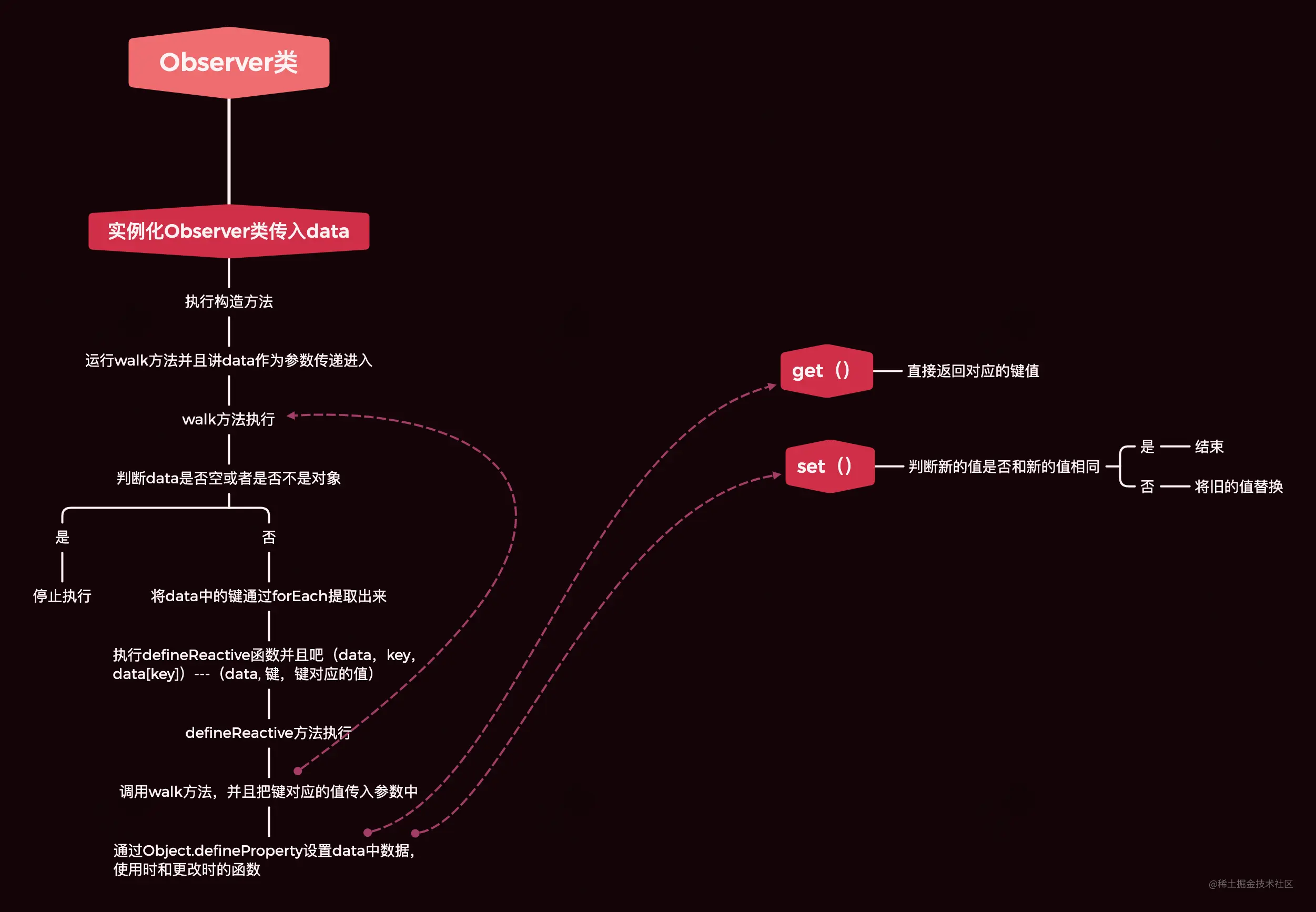There is no operation to change the view , Don't worry, because there are three classes to write , We are now Vue Use... In this class Observer Quasi good logging ～

``````import {Observer} from './Observer'

class Vue {
constructor(option) {
this.\$el = option.el ?
document.querySelector(option.el)
:
document.querySelector('#app')

this.\$data = option.data()

this._proxyData(this.\$data)

new Observer(this.\$data)
}

_proxyData(data) {
Object.keys(data).forEach(key => {
Object.defineProperty(this, key, {
get() {
return data[key]
},
set(newValue) {
if(newValue === data[key]) return

data[key] = newValue
}
})
})
}
}
Copy code ``````

That's good. , I've written the logic for a while, but I can't see the actual effect , Now let's present a compiler（ The compiler is ready , Let's see the page directly ）

4. compiler Compiler class

• Realize to dom Compilation of text nodes
• Created in the root directory `Compiler.js` file
``````export class Compiler {
constructor(vm) {
this.vm = vm
this.el = vm.\$el

this.compile(this.el)
}

compile(el) {
//  Get all the child nodes of the element
let childNodes = [...el.childNodes]
childNodes.forEach(node => {
//  If it is a text node
if(this.isTextNode(node)) {
this.compileText(node)

//  If it's an element node
} else if(this.isElementNode) {

}

//  Determine whether there are any child nodes under the element , If so, recursion
if(node.childNodes && node.childNodes.length) {
this.compile(node)
}

})
}
compileText(node) {
let val = node.textContent
let reg = /\{\{(.+?)\}\}/

if(reg.test(val)) {
//  Take the value inside the double braces
let key = RegExp.\$1.trim()
node.textContent = val.replace(reg, this.vm[key])
}
}

compileElement(node) {
//  There's no need to write anything here first
}

isTextNode(node) {
return node.nodeType === 3
}

isElementNode(node) {
return node.nodeType === 1
}

}
Copy code ``````

I still have to wait until I write a flowchart , wait a moment ！

ok, Again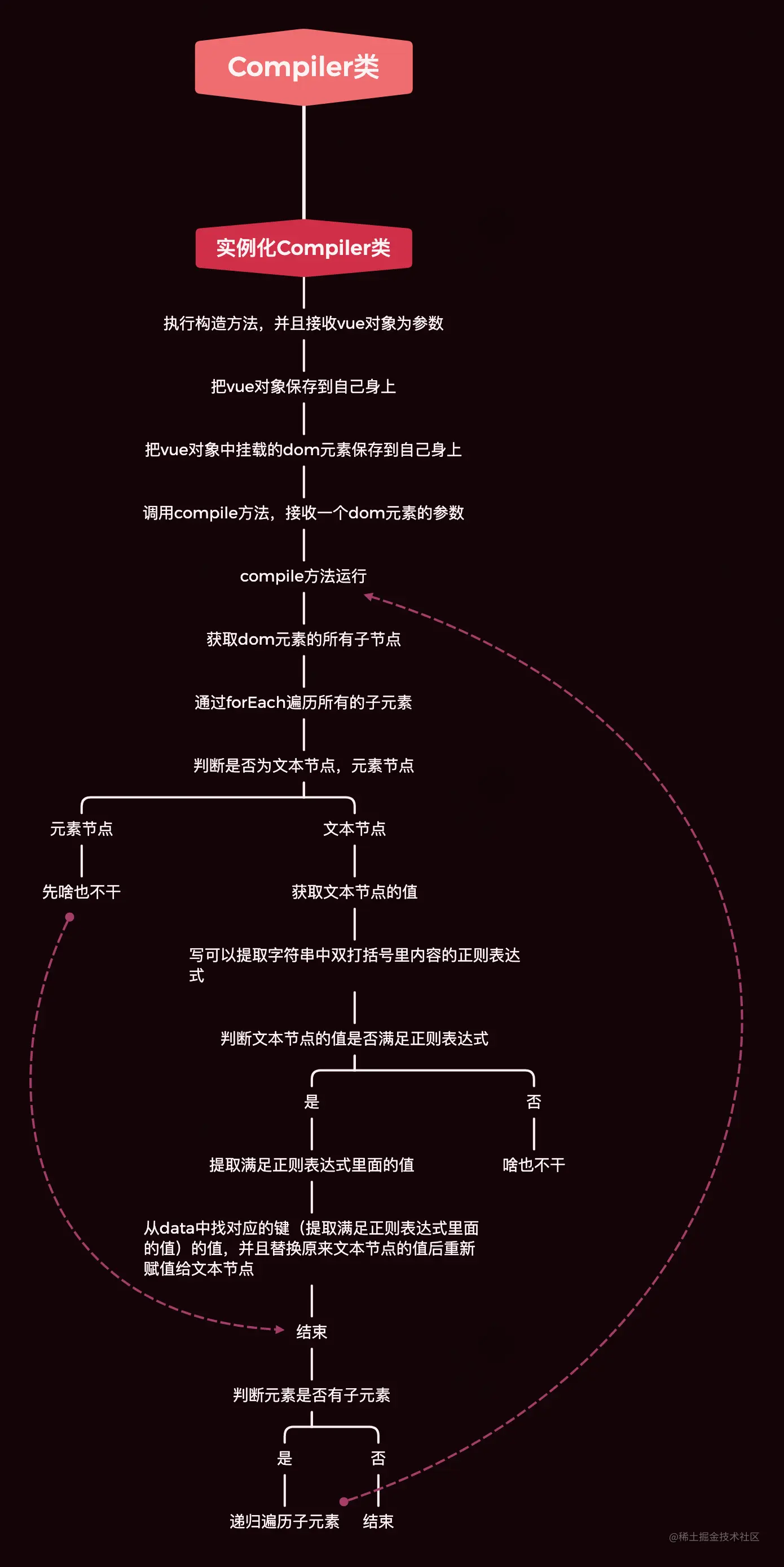Hey, hey, at this time, we can see the effect immediately ！！！！！！！！！

We are index.html That's what it says in ！

``````<div id="app">
{{name}}
</div>

<script type="module" src="./index.js"></script>
Copy code ``````

stay Vue Class Compiler Class and , And write that

``````import {Observer} from './Observer'
import {Compiler} from './Compiler'

class Vue {
constructor(option) {
this.\$el = option.el ?
document.querySelector(option.el)
:
document.querySelector('#app')

this.\$data = option.data()

this._proxyData(this.\$data)

new Observer(this.\$data)

new Compiler(this) //  Use it
}

_proxyData(data) {
Object.keys(data).forEach(key => {
Object.defineProperty(this, key, {
get() {
return data[key]
},
set(newValue) {
if(newValue === data[key]) return

data[key] = newValue
}
})
})
}
}
//  So write it here
new Vue({
data() {
return {
name: ' I am a console No, log'
}
}
})
Copy code ``````

Now look at our page ！！！！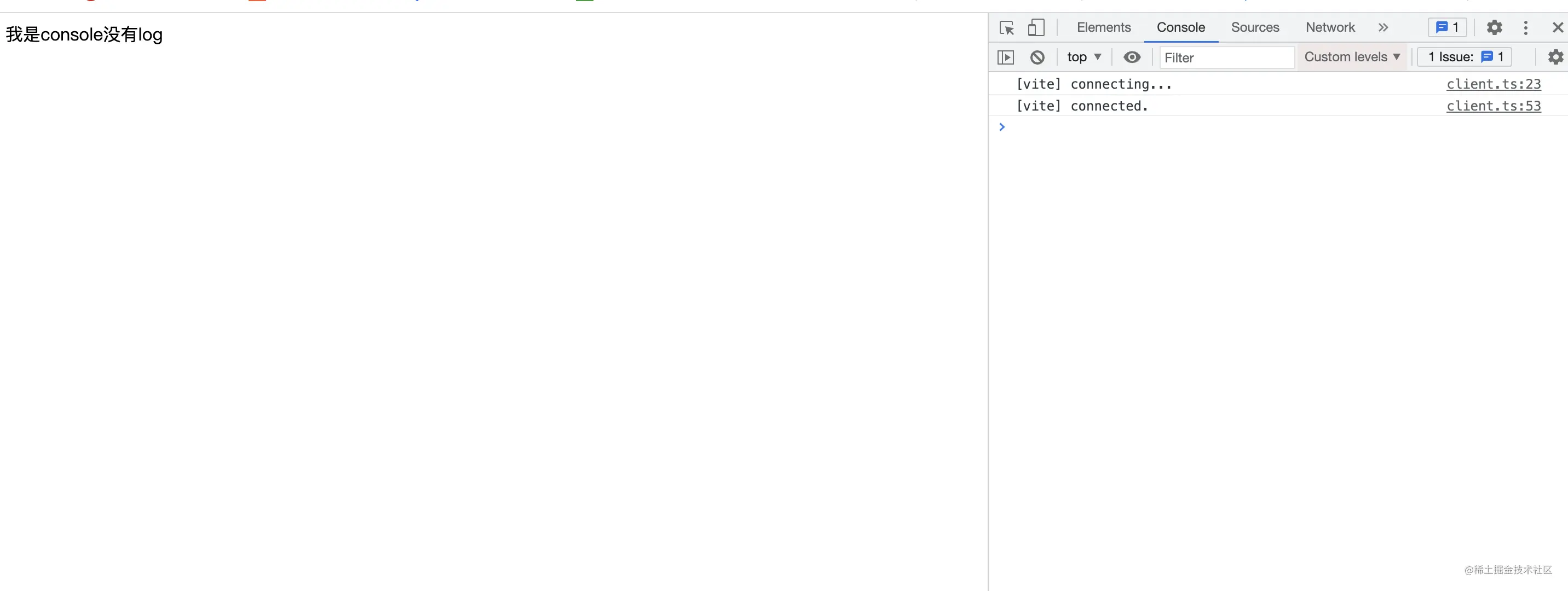That's a good thing , Really great

The following two classes are a little troublesome to explain , My solution is , I'm going to write these two classes at one go , Draw a whole flow chart directly ！！ Directly understand .

5. Dep class

• Created in the root directory `Dep.js`
• use `addSub` Methods can be found in the current `Dep` Add a... To the object `Watcher` Subscription operation of ;
• use `notify` Method notification current `Dep` Object's `subs` All in `Watcher` Object triggers the update operation
``````export class Dep {
constructor() {
this.subs = []
}

if(sub && sub.update) {
this.subs.push(sub)
}
}

notify() {
this.subs.forEach(sub => {
sub.update()
})
}
}
Copy code ``````
6. watcher class

• Created in the root directory `Watcher.js`
• Its function is to , Add a listener to each variable used on the page , whenever data Data changes in , It will receive a notification , This updates the page . If the variable is not used on the page , Then let him bind the view update method, which is not a waste . ---- ` Please pay attention to this sentence `
``````
import {Dep} from './Dep'

export class Watcher {
constructor(vm, key, cd) {
this.vm = vm
this.key = key
this.cd = cd
Dep.nb = this

this.oldValue = vm[key]

Dep.nb = null

}

update() {
let newValue = this.vm[this.key]

if(newValue === this.oldValue) return

this.cd(newValue)
}
}
Copy code ``````

Now let's use these two classes

watcher be used Compiler in

``````import { Watcher } from "./Watcher"

export class Compiler {
constructor(vm) {
this.vm = vm
this.el = vm.\$el

this.compile(this.el)
}

compile(el) {
let childNodes = [...el.childNodes]
childNodes.forEach(node => {

if(this.isTextNode(node)) {
this.compileText(node)
} else if(this.isElementNode) {

}

if(node.childNodes && node.childNodes.length) {
this.compile(node)
}

})
}
compileText(node) {
let val = node.textContent
let reg = /\{\{(.+?)\}\}/

if(reg.test(val)) {
let key = RegExp.\$1.trim()
node.textContent = val.replace(reg, this.vm[key])

new Watcher(this.vm, key, (newValue) => { //  Here it is  --  We will set the method of updating the page for the variables used by the page
node.textContent = newValue
})
}
}

compileElement(node) {
//  There's no need to write anything here first
}

isTextNode(node) {
return node.nodeType === 3
}

isElementNode(node) {
return node.nodeType === 1
}

}
Copy code ``````

Dep be used Observer Inside

``````import { Dep } from "./Dep"

export class Observer {
constructor(data) {
this.walk(data)
}

walk(data) {
if(!data || typeof data !== 'object') return
Object.keys(data).forEach(key => {
this.defineReactive(data, key, data[key])
})
}

defineReactive(obj, key, value) {
const self = this
self.walk(value)
let dep = new Dep() //  here
Object.defineProperty(obj, key, {
get() {
return value
},
set(newValue) {
if(newValue === value) return
value = newValue
self.walk(value)
dep.notify() //  here
}
})

}
}
Copy code ``````

Look at this theory again , Then look at the whole flow chart

First, in the `observer` In the process of registration `get` Method , This method is used for 「 Rely on collection 」. There will be a... In its closure `Dep` object , This object is used to store Watcher Instance of object . Actually 「 Rely on collection 」 The process is to turn `Watcher` The instance is stored in the corresponding `Dep` Go to the object .`get` Methods can make the current `Watcher` object （Dep.target） Stored in its subs in （`addSub`） Method , When the data changes ,`set` Would call `Dep` Object's `notify` Method to notify it of all internal `Watcher` Object for view update .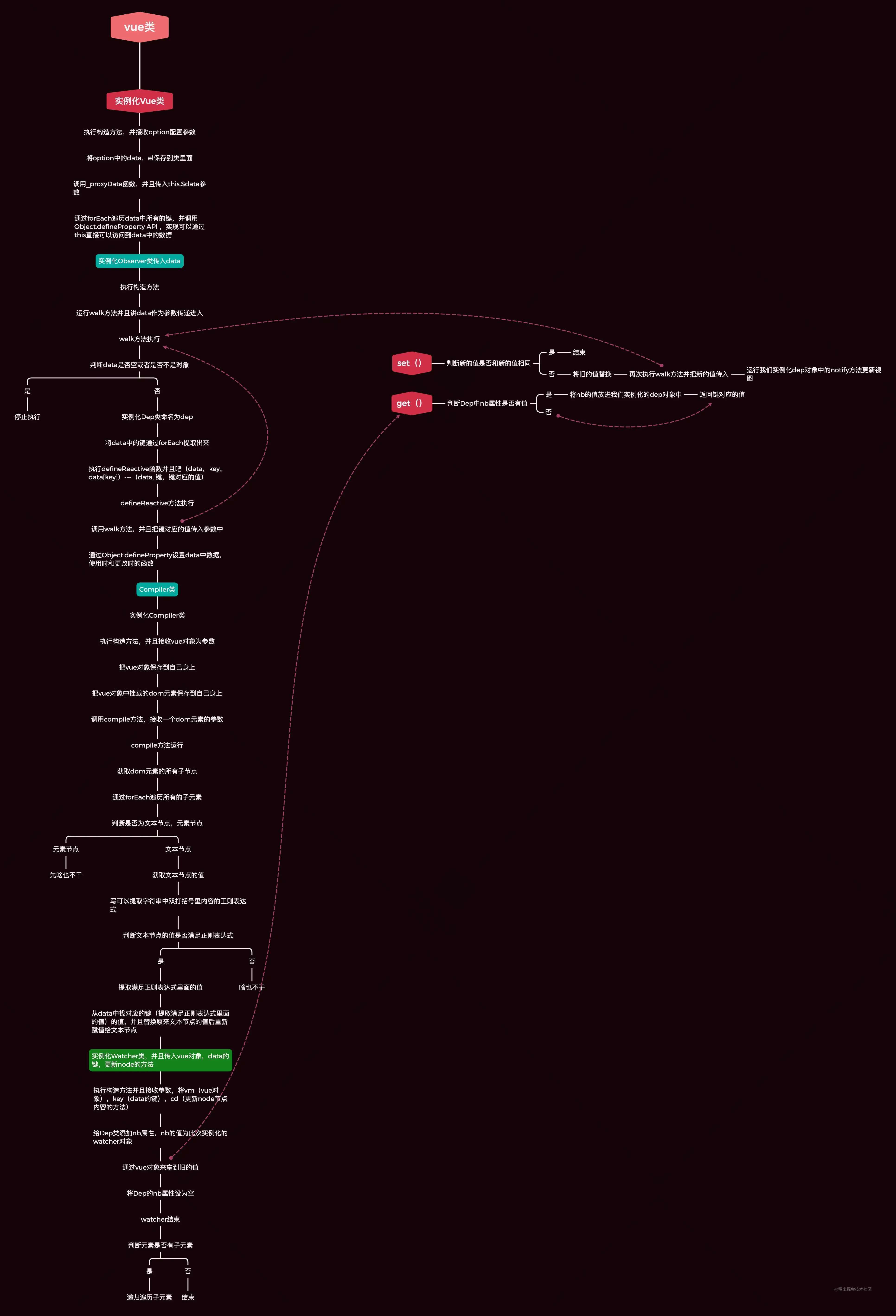A reminder is , If data It will run when the data in the changes Observer Bound in set Method , You can look at it in combination with my flow chart

## Have a play

At this point, the principle that data affects the view is over , After knocking on the code for such a long time , We need to bind events ourselves , To change data Data in , I can't bear it , Now let's play

Remember when we judged that if it was an element node, there was nothing to do , Then we'll do something

``````import {Observer} from './Observer'
import {Compiler} from './Compiler'

class Vue {

constructor(option) {
this.\$el = option.el ?
document.querySelector(option.el)
:
document.querySelector('#app')

this.\$data = option.data()

this.\$methods = option.methods ? option.methods : {}
this._proxyData(this.\$data)
this._initMethods(this.\$methods)
this._proxyMethods(this.\$methods)

new Observer(this.\$data)
new Compiler(this)
}

_proxyData(data) {
Object.keys(data).forEach(key => {
Object.defineProperty(this, key, {
get() {
return data[key]
},
set(newValue) {
if(newValue === data[key]) return
data[key] = newValue
}
})
})
}
_proxyMethods(methods) {
Object.keys(methods).forEach(key => {
Object.defineProperty(this, key, {
get() {
return methods[key]
},
set(newValue) {
if(newValue === methods[key]) return;
methods[key] = newValue
}
})
})
}
_initMethods(methods) {
Object.keys(methods).forEach(key => {
methods[key] = methods[key].bind(this)
})
}
}

Copy code ``````

add to Compiler Medium compileElement Method

``````compileElement(node) {
let self = this,
props = node.getAttributeNames(),
reg = /^c-(\w+)/,
reg2 = /\((.+)\)/,
reg3 = /\'(.+)\'/,
reg4 = /^\d+\$/,
reg5 = /(\w+)\(/,
value,
methodName
props.forEach((key) => {
if (reg.test(key)) {
methodName = RegExp.\$1.trim()
}

let qian = node.getAttribute(key)

if (reg2.test(qian)) {
value = RegExp.\$1.trim()
if (reg4.test(value)) {
value = parseInt(value)
}
if (reg3.test(value)) {
value = RegExp.\$1.trim
}
}

if (reg5.test(qian)) {
qian = RegExp.\$1.trim
}
console.log(qian)
console.log(methodName)
console.log(value)
self.vm[qian](value ? (self.vm[value] ? self.vm[value] : value) : e)
})
})
}

Copy code ``````

Now we can instantiate Vue Object stay index.js Add to file

``````let vue = new Vue({
data() {
return {
name: ' I am a console No, log'
}
},
methods: {
run() {
this.name = ' It's really great '
}
}
})
Copy code ``````

``````<div id="app">
<div>
{{name}}
</div>
<button c-click="run"> change </button>
</div>

<script type="module" src="./index.js"></script>
Copy code ``````

## Conclusion

This is my first article in Nuggets , In fact, I've been struggling with the first article for a long time , I don't know what to send , If you want to send this, you can see that the blogger has written it well , Ah, a little low self-esteem . But I thought again, does everyone study differently , I don't know. I can understand it. Hey, hey

I would like to thank Peng Ge for his guidance , Really helped me a lot , And he's really nice . This is Pengo's Nuggets address , I hope you will pay more attention to Peng ge , It's really powerful

If you like my style of articles, please praise and pay attention to , And comments tell me ！！

Bye ～ See you next time